# Free Vibrations of a Compressor BladeThis test lets you check analysis free vibrations results for a compressor blade in the context of a modal (or frequency) case. You will use 2D meshes.Reference:

• OLSON,M.D.,LINDBERG,G.M., Vibration analysis of cantilevered curved plates using a new cylindrical shell finite element, 2nd Conf. Matrix Methods in Structural Mechanics, WPAFB, Ohio 1968.
• OLSON,M.D.,LINDBERG,G.M., Dynamic analysis of shallow shells with a doubly curved triangular finite element, JSV, Vol. 19, No 3, pp 299-318, 1971.

##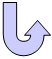Specifications

### Geometry Specifications

 Length: L = 304.8 mm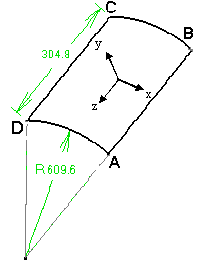Radius: R = 609.6 mm Thickness: th = 3.048 mm Angle: A = 28.6479 deg   (or 0.5 rad)

### Analysis Specifications

 Young Modulus (material): E = 2.0685e+011 N_m2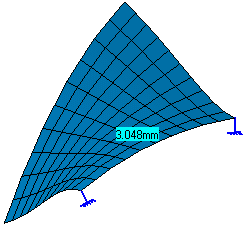Poisson's Ratio (material): ν = 0.3 Density: ρ = 7857.2 Kg/m3 Mesh Specifications: 11 x 11 nodes Restraints: BC straight edge is free AD straight edge is clamped

##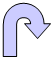Results

The table below presents the frequencies of the first six modes (mesh specifications: 11 x 11 nodes).

 Mode Reference value [Hz] Computed value [Hz] Linear triangle (TR3) Parabolic triangle (TR6) Linear quadrangle (QD4) Parabolic quadrangle (QD8)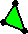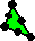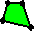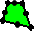1 85.6 84.2461 82.8426 83.6888 85.7079 2 134.5 141.824 136.908 136.16 138.555 3 259.0 248.017 242.163 252.195 245.927 4 351.0 341.879 333.202 337.331 340.94 5 395.0 380.636 373.174 381.633 382.989 6 531.0 538.508 517.818 546.994 527.982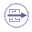To Perform the Test:

The Free_vibrations_compressor_blade_QD8.CATAnalysis document presents a complete analysis of this case, computed with a mesh formed of parabolic and quadrangular elements (QD8).

To compute the case with linear quadrangle (QD4), linear triangle (TR3) and parabolic triangle (TR6) elements, proceed as follow:

1. Open the CATAnalysis document.

2. In the Advanced Meshing Tools workbench, replace the mesh specifications as indicated above.

3. In the Generative Structural Analysis workbench, compute the case.